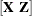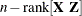#### Containment Degrees of Freedom Approximation

The DDFM=CONTAIN method is carried out as follows: Denote the fixed effect in question as `A` and search the G-side random effect list for the effects that syntactically contain `A`. For example, the effect `B`(`A`) contains `A`, but the effect `C` does not, even if it has the same levels as `B`(`A`).

Among the random effects that contain `A`, compute their rank contributions to thematrix (in order). The denominator degrees of freedom that is assigned to `A` is the smallest of these rank contributions. If no effects are found, the denominator degrees of freedom for `A` is set equal to the residual degrees of freedom,. This choice of degrees of freedom is the same as for the tests performed for balanced split-plot designs and should be adequate for moderately unbalanced designs.

Note: If you have amatrix with a large number of columns, the overall memory requirements and the computing time after convergence can be substantial for the containment method. In this case, you might want to use a different degrees-of-freedom method, such as DDFM=RESIDUAL, DDFM=NONE, or DDFM=BETWITHIN.